# 循环神经网络

Posted by Masutangu on September 12, 2022

• 文本翻译：输入是连续的，需要结合前文进行翻译
• 股价：需要结合昨天的股价进行预测

# 自回归模型

$P(x_t \mid x_{t-1}, \ldots, x_1) = P(x_t \mid f(x_{t-1}, \ldots, x_1))$

$P(x_t \mid x_{t-1}, \ldots, x_1) =P(x_t \mid f(x_{t-1}, \ldots, x_{t-\tau}))$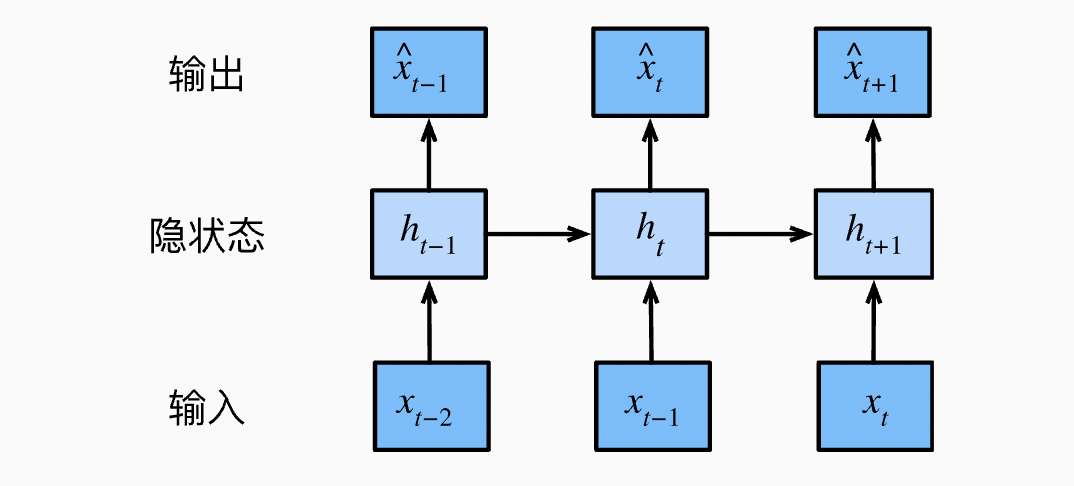# 马尔科夫模型

$P(x_1, \ldots, x_T) = \prod_{t=1}^T P(x_t \mid x_{t-1}, ...,x_1)$

$P(x_1, \ldots, x_T) = \prod_{t=1}^T P(x_t \mid x_{t-1})$

# 循环神经网络 RNN

$P(x_t \mid x_{t-1}, \ldots, x_1) \approx P(x_t \mid h_{t-1})$

$h_t = f(x_{t}, h_{t-1})$

## 无隐状态的神经网络

$\mathbf{H} = \phi(\mathbf{X} \mathbf{W}_{xh} + \mathbf{b}_h)$

$\mathbf{O} = \mathbf{H} \mathbf{W}_{hq} + \mathbf{b}_q$

## 有隐状态的神经网络

$\mathbf{H}_t = \phi(\mathbf{X}_t \mathbf{W}_{xh} + \mathbf{H}_{t-1} \mathbf{W}_{hh} + \mathbf{b}_h)$

$\mathbf{O}_t = \mathbf{H}_t \mathbf{W}_{hq} + \mathbf{b}_q$

1. 拼接当前时间步$t$的输入$\mathbf{X}_t$和前一时间步$t-1$的隐状态$\mathbf{H}_{t-1}$

2. 将拼接的结果送入带有激活函数 $\phi$ 的全连接层，全连接层的输出是当前时间步 $t$ 的隐状态 $\mathbf{H}_t$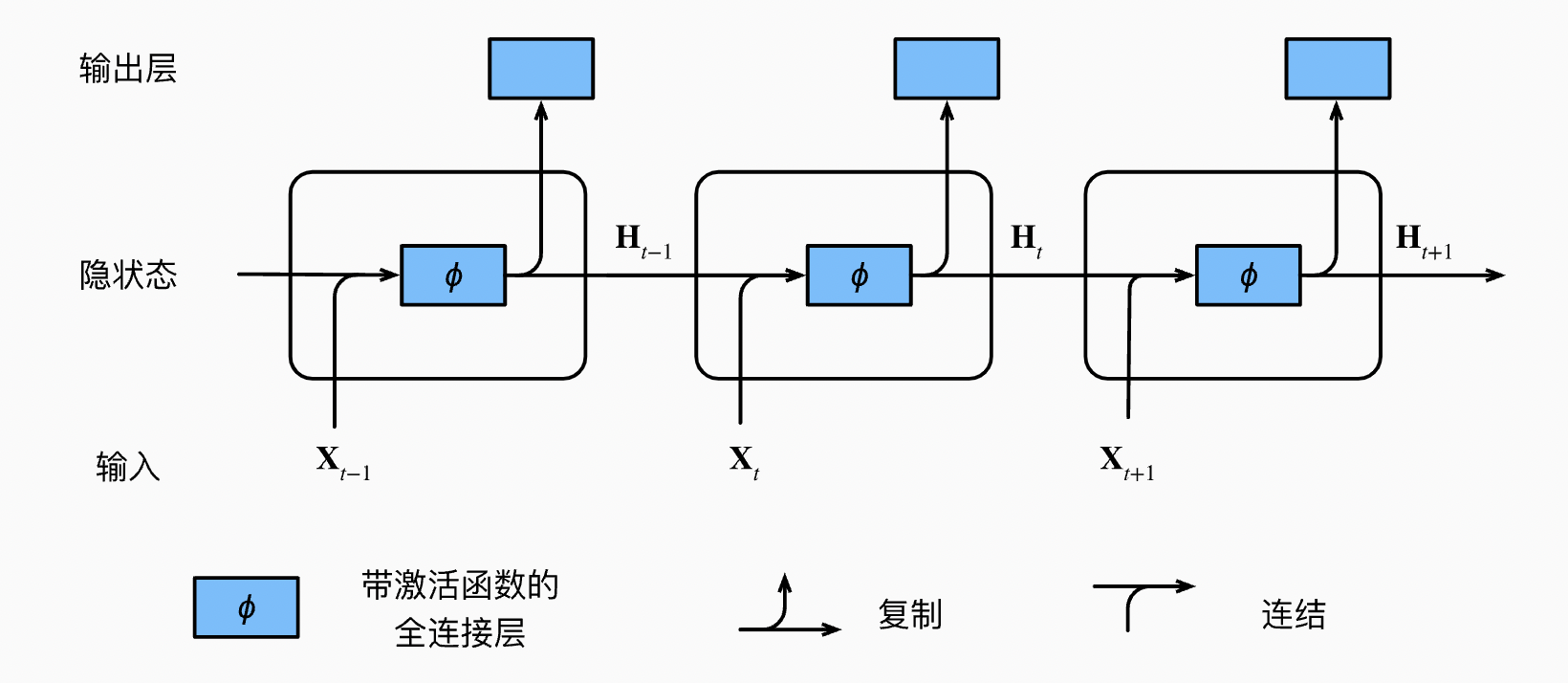# 循环神经网络的梯度分析

\begin{aligned}h_t &= f(x_t, h_{t-1}, w_h)\\o_t &= g(h_t, w_o)\end{aligned}

$L(x_1, \ldots, x_T, y_1, \ldots, y_T, w_h, w_o) = \frac{1}{T}\sum_{t=1}^T l(y_t, o_t)$

\begin{aligned}\frac{\partial L}{\partial w_h} & = \frac{1}{T}\sum_{t=1}^T \frac{\partial l(y_t, o_t)}{\partial w_h} \\& = \frac{1}{T}\sum_{t=1}^T \frac{\partial l(y_t, o_t)}{\partial o_t} \frac{\partial g(h_t, w_o)}{\partial h_t} \frac{\partial h_t}{\partial w_h}\end{aligned}

\begin{aligned}\frac{\partial h_t}{\partial w_h} & = \frac{\partial f(x_{t},h_{t-1},w_h)}{\partial w_h} +\frac{\partial f(x_{t},h_{t-1},w_h)}{\partial h_{t-1}} \frac{\partial h_{t-1}}{\partial w_h} \\ & =\frac{\partial f(x_{t},h_{t-1},w_h)}{\partial w_h}+\sum_{i=1}^{t-1}\left(\prod_{j=i+1}^{t} \frac{\partial f(x_{j},h_{j-1},w_h)}{\partial h_{j-1}} \right) \frac{\partial f(x_{i},h_{i-1},w_h)}{\partial w_h}\end{aligned}

# 长短期记忆网络

## 输入门、忘记门和输出门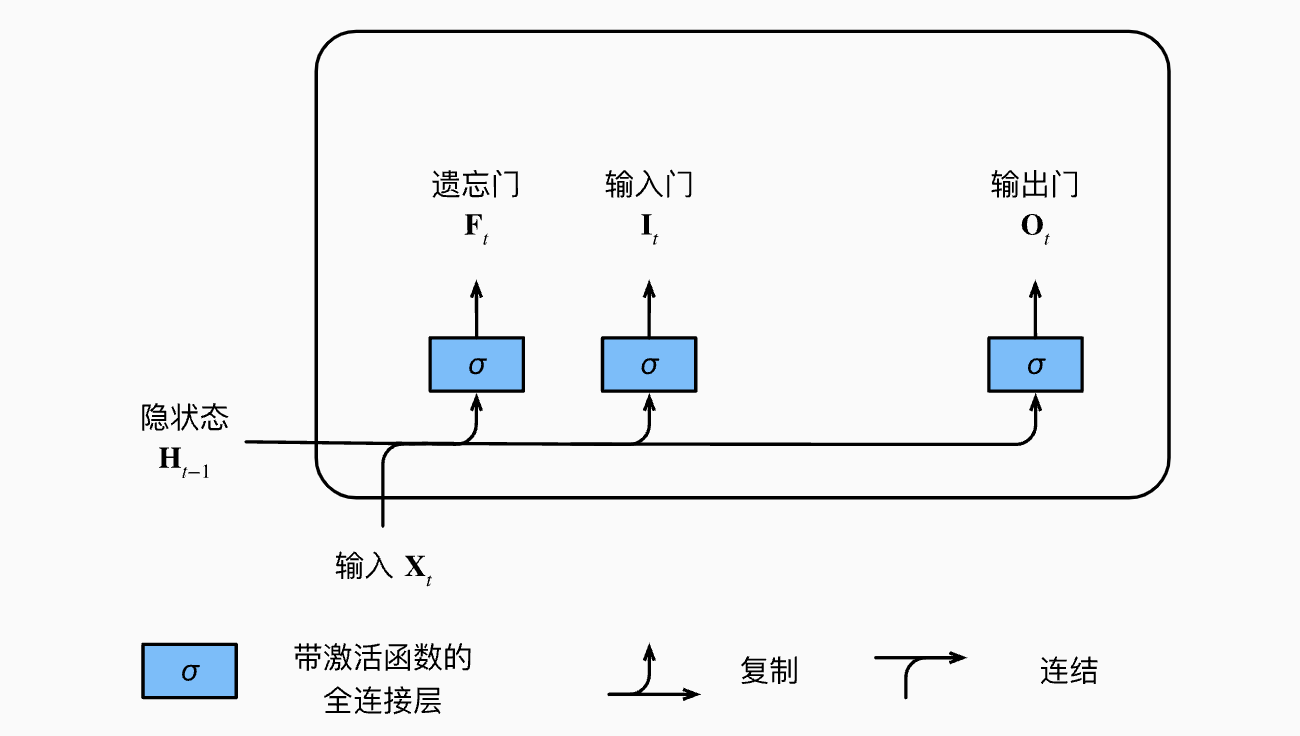\begin{aligned} \mathbf{I}_t &= \sigma(\mathbf{X}_t \mathbf{W}_{xi} + \mathbf{H}_{t-1} \mathbf{W}_{hi} + \mathbf{b}_i)\\ \mathbf{F}_t &= \sigma(\mathbf{X}_t \mathbf{W}_{xf} + \mathbf{H}_{t-1} \mathbf{W}_{hf} + \mathbf{b}_f)\\ \mathbf{O}_t &= \sigma(\mathbf{X}_t \mathbf{W}_{xo} + \mathbf{H}_{t-1} \mathbf{W}_{ho} + \mathbf{b}_o) \end{aligned}

## 候选记忆元和记忆元

$\tilde{\mathbf{C}}_t = \text{tanh}(\mathbf{X}_t \mathbf{W}_{xc} + \mathbf{H}_{t-1} \mathbf{W}_{hc} + \mathbf{b}_c)$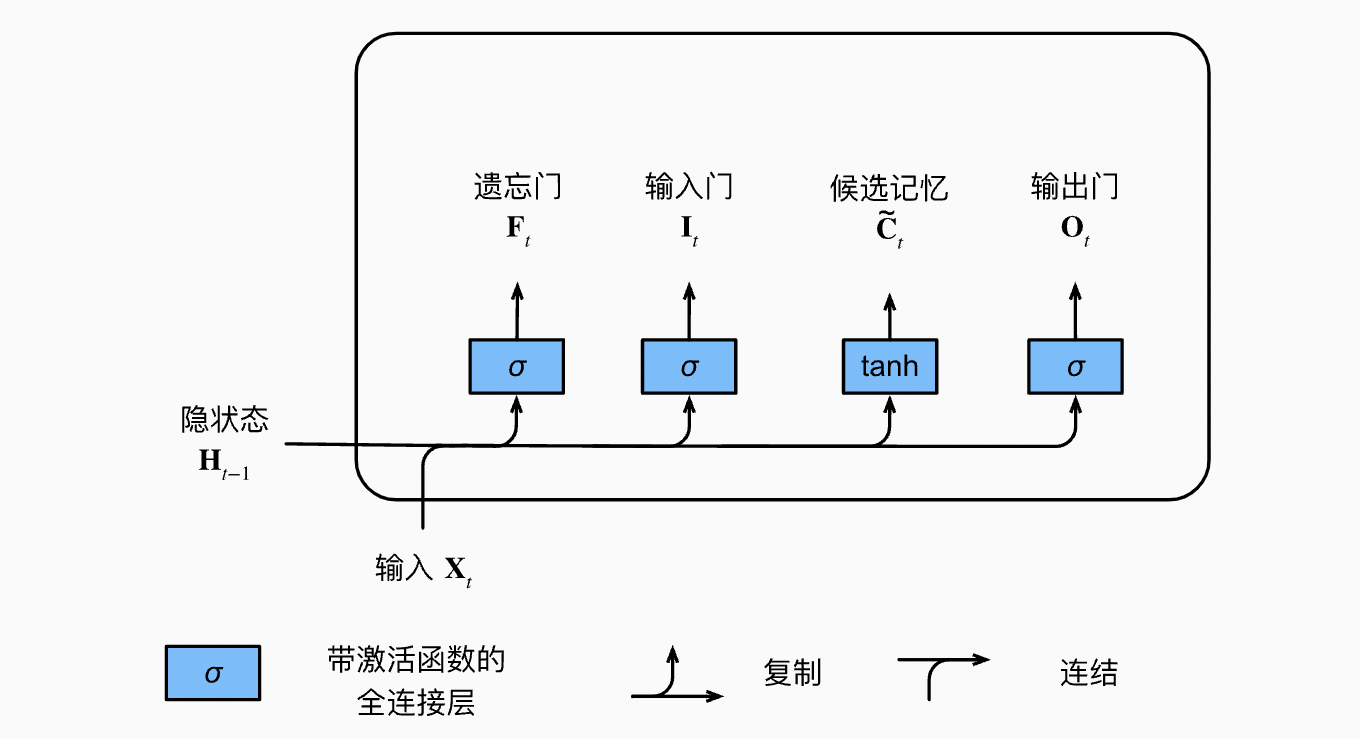$\mathbf{C}_t = \mathbf{F}_t \odot \mathbf{C}_{t-1} + \mathbf{I}_t \odot \tilde{\mathbf{C}}_t$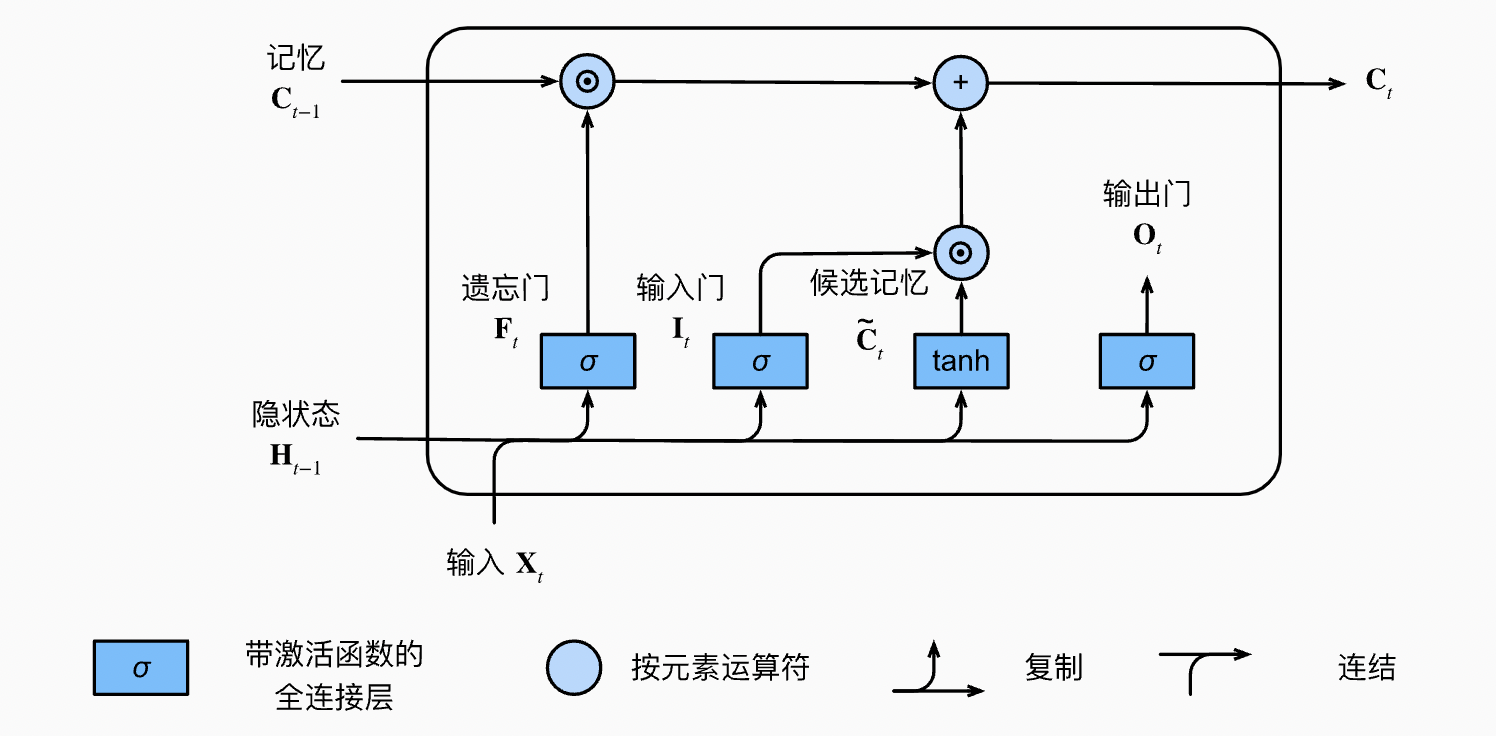## 隐状态

$\mathbf{H}_t = \mathbf{O}_t \odot \tanh(\mathbf{C}_t)$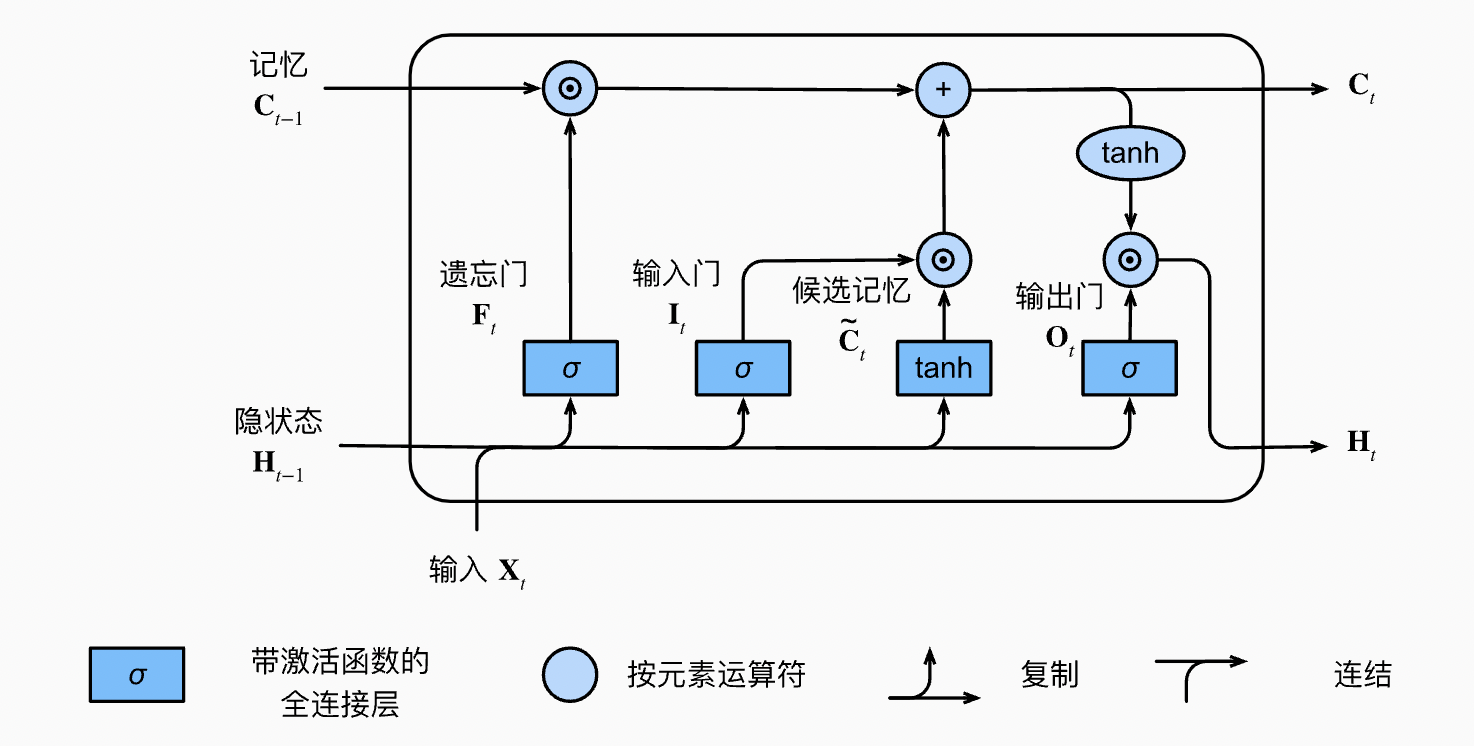# 深度循环神经网络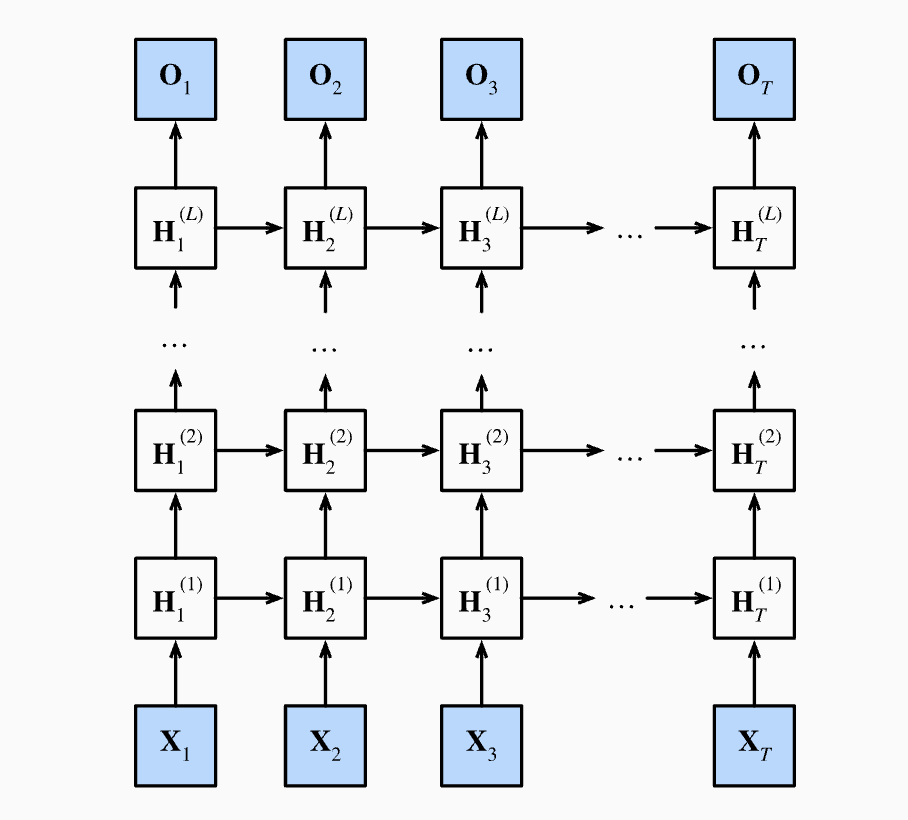$\mathbf{H}_t^{(l)} = \phi_l(\mathbf{H}_t^{(l-1)} \mathbf{W}_{xh}^{(l)} + \mathbf{H}_{t-1}^{(l)} \mathbf{W}_{hh}^{(l)} + \mathbf{b}_h^{(l)})$

$\mathbf{O}_t = \mathbf{H}_t^{(L)} \mathbf{W}_{hq} + \mathbf{b}_q$

# 参考文献